# Scanner - Revenue Notes | Study Economics Class 11 - Commerce

## Commerce: Scanner - Revenue Notes | Study Economics Class 11 - Commerce

The document Scanner - Revenue Notes | Study Economics Class 11 - Commerce is a part of the Commerce Course Economics Class 11.
All you need of Commerce at this link: Commerce

N.C.E.R.T Question’s

(Q1) Why is the total revenue curve facing a competitive firm a straight line passing through origin

(Q2) How does the TR change with output when MR is positive ?

(Q3) A perfectly competitive firm faces market price equal to Rs.15 .

(a) Derive its TR schedule for the range of output from 0 to 10 units
(b) Suppose the market price increases to Rs.17. Will the new TR curve be flatter or steeper ?

Q4) What is the relationship between Market price and MR of a price taking firm ?

C.B.S.E Question’s

(Q1) Which concept of revenue is called price ?

(Q2) What changes in TR will result in (i) A decrease in MR (ii) An increase in MR

(Q3) What will happen to MR when (i) TR increases at a decreasing rate (ii) TR increases at constant rate ?

(Q4) Define MR, AR, TR ?

(Q5) Complete the table and Also plot the curves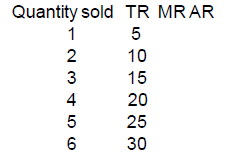CBSE 2008 + Sample Paper Questions

(Q1) Define marginal revenue. State the relation between marginal revenue and average revenue when a firm :

(i) is able to sell more quantity of output at the same price.
(ii) is able to sell more quantity of output only by lowering the price. (3)

(Q2) Complete the following table: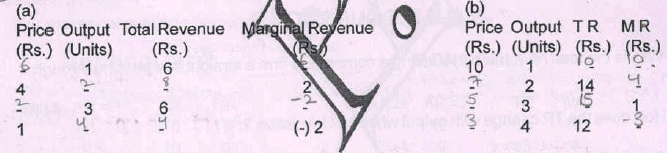(Q3) Complete the following table: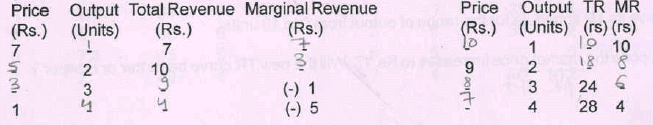CBSE 2009

(Q1) State true or false with reasons ::

(i) When marginal revenue is positive and constant, average and total revenue will both
increase at constant rate.

(ii) When total revenue is maximum, marginal revenue is also maximum.

(Q2) Complete the following table :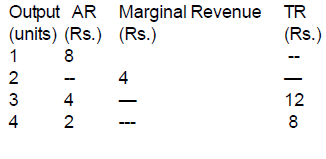(Q3) Complete the following table :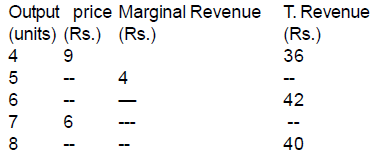CBSE 2010 + Sample Paper Questions

(Q1) State true or false. Give reasons for your answer : When marginal revenue is contant and not equal to zero, then total revenue will also be constant.

(Q2) Explain the relation between marginal revenue and average revenue when a firm is able to
sell more quantity of output (4 M)

(i) at the same price.
(ii) only by lowering the price.

CBSE 2011 & CBSE 2012

(Q1) Draw in a single diagram the average revenue and marginal revenue curves of a firm which can sell any quantity of the good at a given price. Explain. (3 marks)
OR
Explain the relation between average revenue and marginal revenue of a firm which is free to sell any quantity at a given price. (3 marks)

(Q2) What is the behaviour of Marginal Revenue in a market in which a firm can sell any quantity of the output it produces at a given price ? (1 mark)

(Q3) State True or false

(i) When MR is zero , AR will be constant
(ii) MR is always the price at which the last unit of a commodity is sold   CBSE (D)

SAMPLE PAPER 2014-15 & 15-16 & 16-17

(Q1) State, with valid reasons, which of the following statement are true or false: (a) Average Revenue curve under the Perfect Competition is a downward sloping curve. (b) When MR is falling but positive, TR will also be falling and positive. (6)

Ans :: (a) False: Since the firm under Perfect Competition is a price taker , AR curve will be a straight line parallel to X-axis. (b) False: When MR is falling but positive, TR will be rising.  (brief explanation of each)

C.B.S.E  2013

(Q1) Define marginal revenue. (1 M)

Ans :: It is the addition to total revenue when sales are increased by one unit.

(Q2) When will Marginal Revenue be negative ?

Ans :: When increase in sale by one more unit results in fall in TR.

C.B.S.E Paper 2014

(Q1) Why is average Revenue always equal to price ? (3M)

(Q2) Define marginal revenue. (1 M)

Ans :: Addition to total revenue on selling or producing one more unit of output is marginal revenue.

(Q3) How is marginal revenue (MR) calculated ? (1 M)

(Q4) What is meant by Revenue in microeconomics ?

Ans :: Receipts from sale of a good or market value of the output produced is called revenue

(Q5) State the relationship between average and marginal revenue ?

(Q6) Under what market condition does average revenue always equal to marginal revenue ?

Ans :: It is under the market condition when a firm can sell more at the given price that AR = MR throughout as production is increased by the firm. It is because the firm is a price taker. It means that price, which is same as AR, remains unchanged throughout.
By the average – marginal relationship, AR remains unchanged only when AR = MR throughout.

C.B.S.E Paper 2015

(Q1) Define Average Revenue. Show that Average Revenue and Price are same.

(Q2) Complete the following table : (D)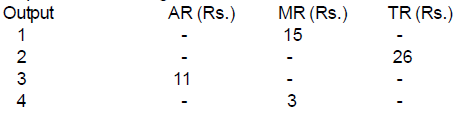(Q3) Why is average revenue curve of a firm parallel to x-axis under perfect competition ?Explain. [AI (C)]

Ans :: Under perfect competition, an individual firm is a price taker and has no influence on market price. The firm is free to sell any quantity at the market determined price. As such the AR curve of a firm is parallel to the X-axis.

(Q4) Why is average revenue curve of a firm negatively sloped under monopolisticcompetition ? Explain. [AI (C)]

Ans :: Under monopolistic competition, a firm enjoys some influence in determining market price of its product. The firm can sell more only by lowering the price. It makes the firm’s AR curve downward sloping

(Q5) Define revenue. State the relation between marginal revenue and average revenue.

Ans :: Revenue in Economics refers to the market value of output produced or receipts from sale of output produced. (1M)

If MR > AR, AR rises

If MR = AR, AR is constant (3M)

If MR < AR, AR Falls. (Diagram not required)

CBSE 2016

(Q1) A firm is able to sell any quantity of a good at a given price. The firm’s marginal revenue will

(a) Greater than Average Revenue

(b) Less than Average Revenue

(c) Equal to Average Revenue

(d) Zero

Ans :: (c) Equal to AR

(Q2) Suppose total revenue is rising at a constant rate as more and more units of a commodity are sold, marginal revenue would be : (choose the correct alternative) (1)

(a) Greater than average revenue

(b) Equal to average revenue

(c) Less than average revenue

(d) Rising

Ans :: (b) Equal to Average Revenue

(Q3) A firm is able to sell more quantity of a good only by lowering the price. The firm’s marginal revenue, as he goes on selling, would be : (choose the correct alternative)

(a) Greater than average revenue

(b) Less than average revenue

(c) Equal to average revenue

(d) Zero

Ans :: (b)Less than AR

The document Scanner - Revenue Notes | Study Economics Class 11 - Commerce is a part of the Commerce Course Economics Class 11.
All you need of Commerce at this link: CommerceUse Code STAYHOME200 and get INR 200 additional OFF

## Economics Class 11

201 videos|195 docs|64 tests

Track your progress, build streaks, highlight & save important lessons and more!

,

,

,

,

,

,

,

,

,

,

,

,

,

,

,

,

,

,

,

,

,

;